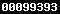### PROFIL PEMECAHAN MASALAH SISWA TUNA GRAHITA PADA PEMBELAJARAN MATEMATIKA DENGAN MENGGUNAKAN PENDEKATAN FORMAL DAN PENDEKATAN FORMAL DIVARIASI PENDEKATAN FUNGSIONAL

#### Sari

Abstract: The objectives of this research were to investigate: (1) the profile of problem solving in mathematics of a deaf child in solving the geometrics problems with the learning using the formal approach; and (2) the profile of problem solving in mathematics of a deaf child in solving the geometrics problems with the learning using the formal approach varied with the functional approach. This research used the qualitative explorative research method. The subject of the research consisted of one deaf male student and was taken by using the purposive sampling technique. The subject was determined according to the following considerations: (1) the student had not too few and not too many incorrect answers; and (2) he could communicate well based on the information obtained from his teacher. The data of the profile of problem solving in mathematics were gathered through test and interview with the student on how to solve the test problems. The data were validated by using the time triangulation. They were analyzed by using the interactive model of analysis consisting of the following measures: data reduction, data display, and conclusion drawing. The results of the research  were as follows: 1) The problem solving in Mathematics of the deaf child with the learning using the formal approach results in the following findings: (a) recognizing some colors when they are the colors of flat structures; (b) being difficult to understand the meanings of questions if they contain more than one answer; (c) being unable to recognize some spatial structures well; (d) being unable to use ruler; and (e) unable to differentiate the letters well. 2) The problem solving in mathematics of the deaf child with the learning using the formal approach varied with the functional results in the following findings: (a) at the beginning the child is difficult to recognize some of the spatial structures, but after having practiced drawing with colors and then compared them with the ones in the spatial structure learning, that is the ones in the test problems and the ones in the learning instruments, he gradually recognizes the spatial structure; (b) principally he can recognize the spatial structures, but when the spatial structures are put vertically and horizontally, he feels difficult to recognize them; (c) the students is difficult to count the number of sides of quadrangle pyramid ; and (d) he is difficult to understand the meanings of questions so that the answers given are not synchronized with the questions.

Keywords : Profile, problem solving, deaf, formal approach, and functional approach

PDF

### Refbacks

• Saat ini tidak ada refbacks.View My Stats